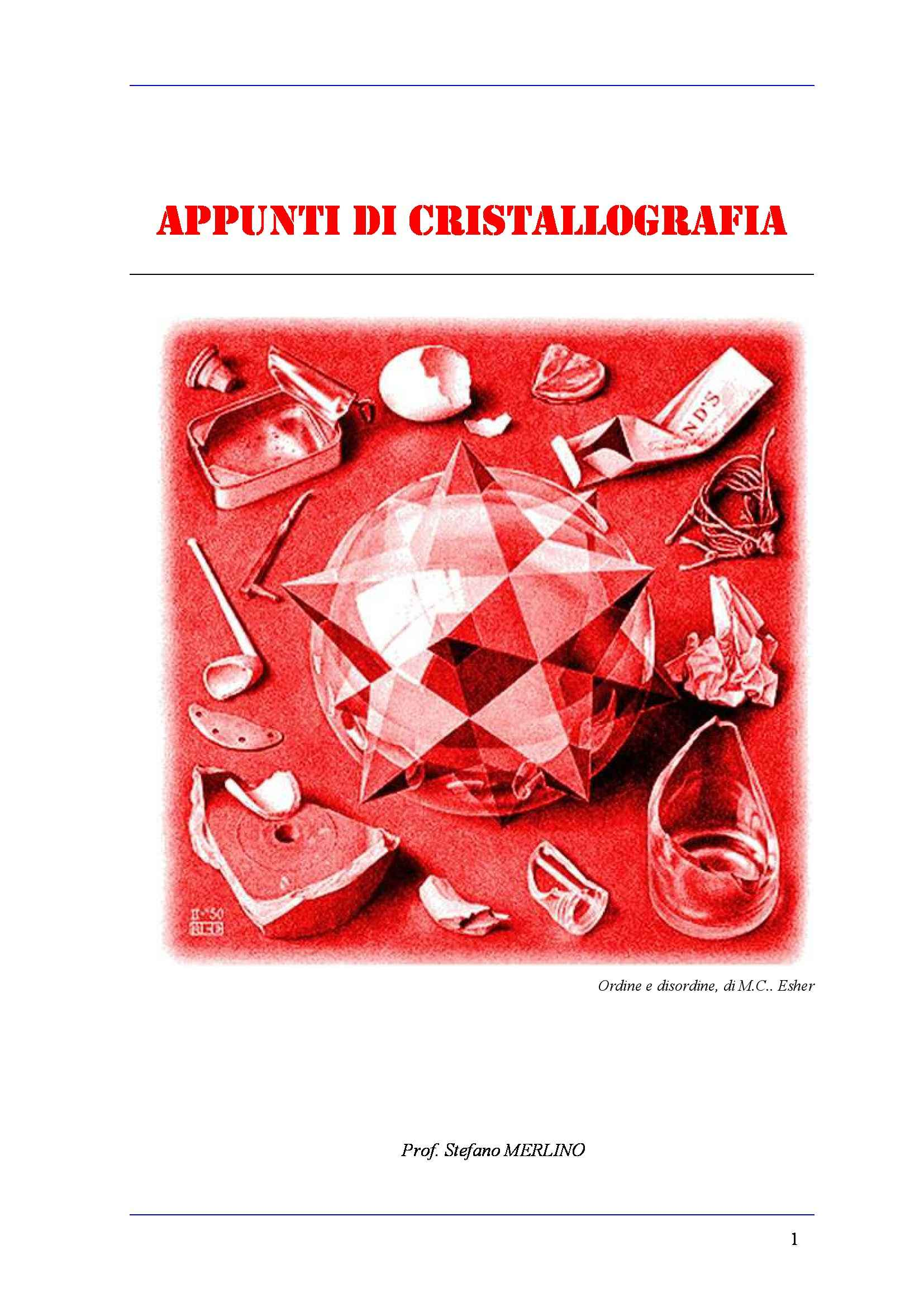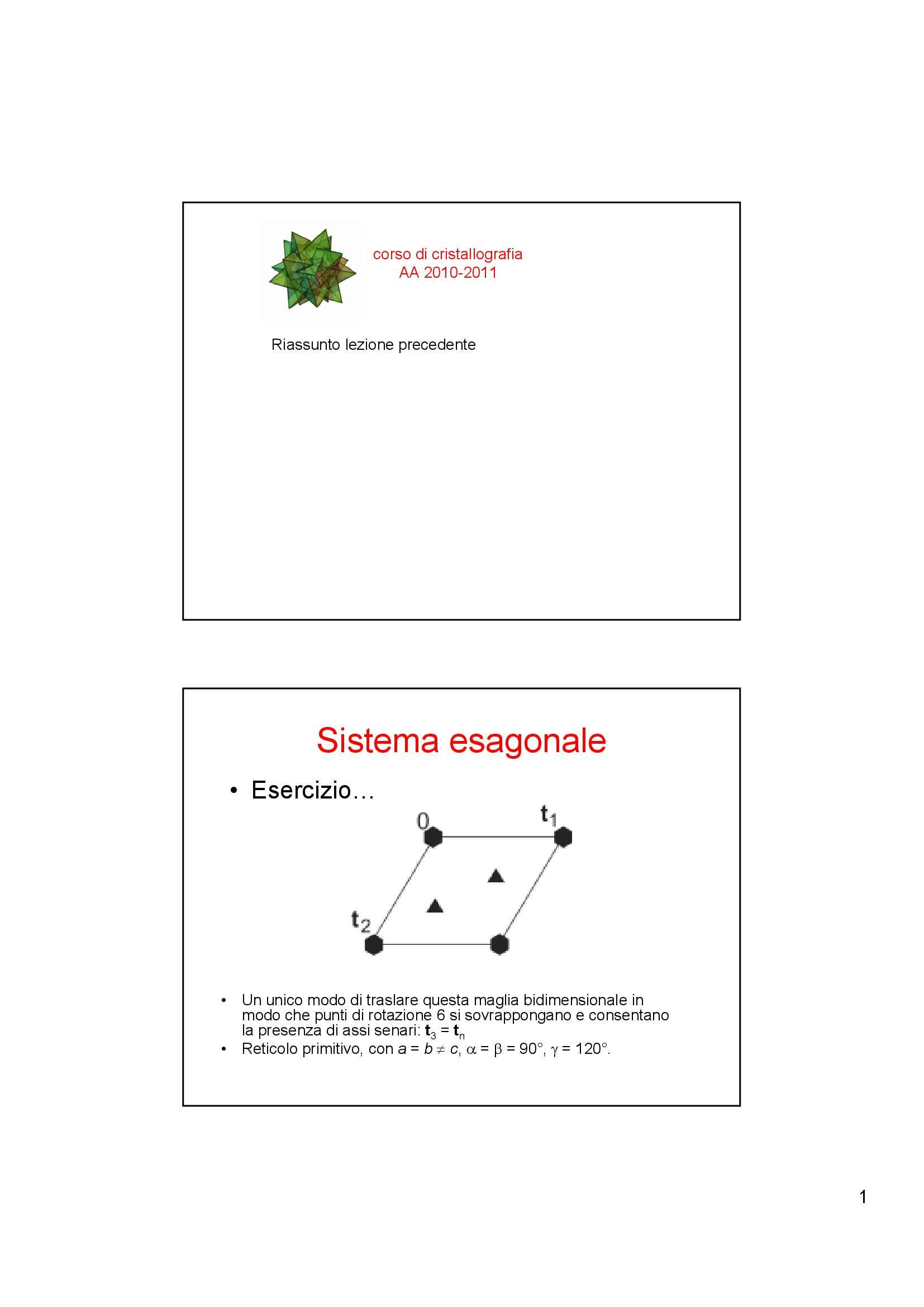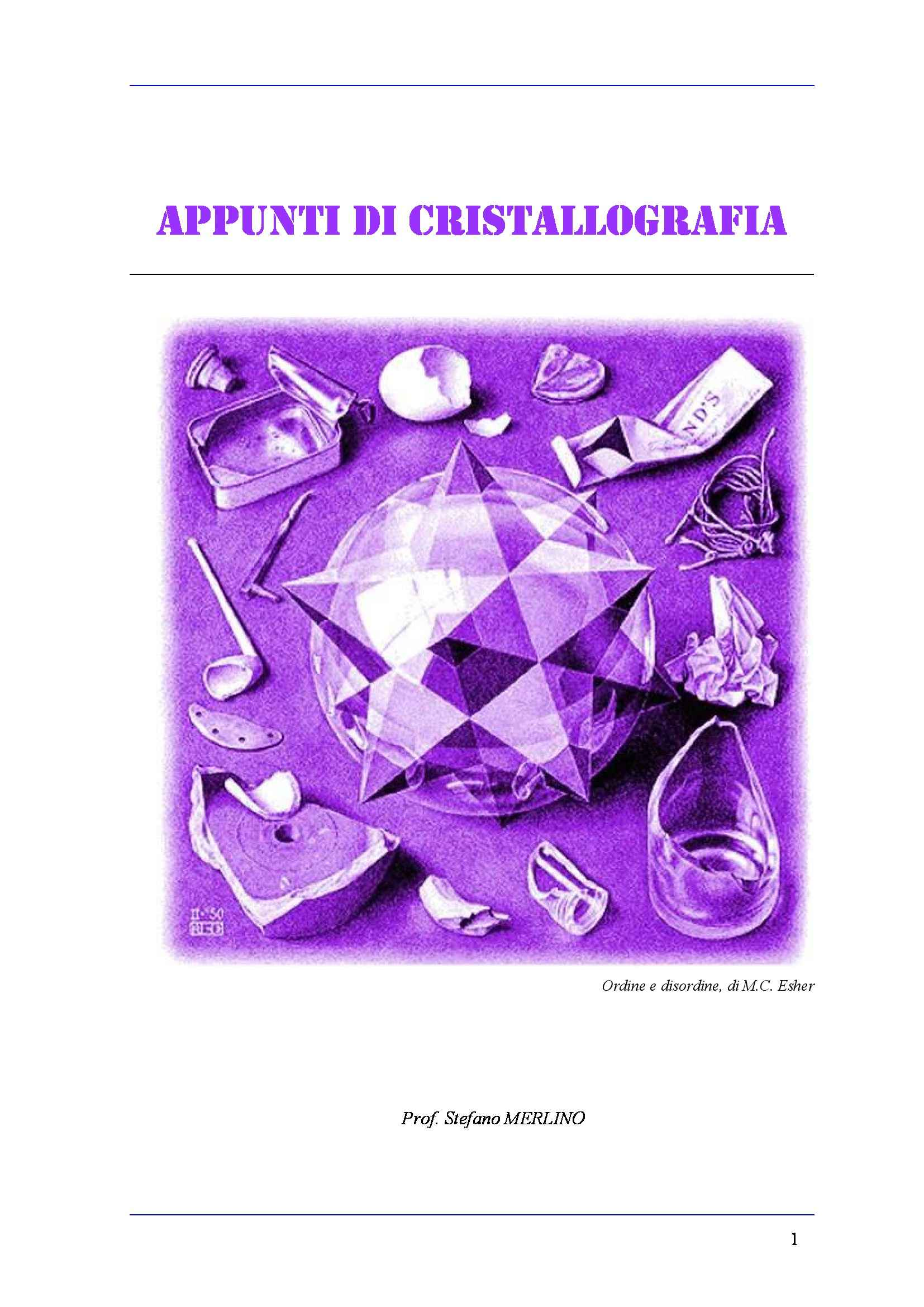Che materia stai cercando?

# Metodi diretti per la determinazione della struttura cristallina - Clegg

Materiale didattico per il corso di Cristallografia della prof.ssa Elena Buonaccorsi. Trattasi del capitolo 10 a cura di W. Clegg del testo dal titolo "Crystal Structure Analysis. Principls and Practice", all'interno del quale è affrontato il tema dei metodi diretti per la determinazione della struttura cristallina. Vedi di più

Esame di Cristallografia docente Prof. E. Bonaccorsi

Anteprima

### ESTRATTO DOCUMENTO

Direct methods of 10

crystal-structure

determination

Peter Main

Many methods of structure determination have been termed ‘direct’

(e.g. Patterson function, Fourier methods) in that, under favourable cir-

cumstances, it is possible to proceed in logical steps directly from the

measured X-ray intensities to a complete solution of the crystal struc-

ture. However, the term ‘direct’ is usually reserved for those methods

that attempt to derive the structure factor phases, electron density or

atomic co-ordinates by mathematical means from a single set of X-ray

intensities. Of these possibilities, the determination of phases is the most

important for small-molecule crystallography.

10.1 Amplitudes and phases

The importance of phases in structure determination is obvious, but it

is instructive to examine their importance relative to the amplitudes. To

do this, we use the convolution theorem, which is set out in Appendix A.

It is not necessary to understand the mathematics in detail, but we are

going to use the same relationship among the functions seen in Section

A.10.

Let us regard a structure factor as the product of an amplitude |F(h)|

h

and a phase factor exp(iφ(h)), where is a reciprocal space vector, the

set of three indices being represented here by a single symbol. We will

call the Fourier transform of the ‘amplitude synthesis’ and the

|F(h)|

Fourier transform of the function exp(iφ(h)) the ‘phase synthesis’. The

convolution theorem gives: F(h),

exp(iφ(h))

|F(h)| × =

## F.T. F.T. F.T.

amplitude synthesis * phase synthesis = electron density

(10.1)

133

Direct methods of crystal-structure determination

134 1

a 1

o 2

× ×

×

×

b ×

×

1

2 A B

Fig. 10.1 Fourier synthesis calculated from exp(iφA), where and are different

|F |

## A B

structures. Atomic positions of structure are marked by dots, those of by crosses.

Nature 190,

Reprinted by permission from Macmillan Publishers Ltd: 161, copyright 1961.

where * is the convolution operator. The amplitude synthesis must look

rather like the Patterson function with a large origin peak, and its convo-

lution with the phase synthesis will put this large peak at the site of each

peak in the phase synthesis. The phase synthesis must therefore contain

peaks at atomic sites for the convolution to give the electron density. It is

thus the phases rather than the amplitudes that give information about

atomic positions in an electron-density map. A good illustration of this

was given by Ramachandran and Srinivasan (1961), who calculated an

electron-density map using the phases from one structure (A) and the

amplitudes from another (B). The map, in Fig. 10.1, shows the electron-

density peaks corresponding to the atomic positions in structure Arather

than B. Clearly, of the two problems Nature could have given us, the

phase problem is much more difficult than the amplitude problem.

10.2 The physical basis of direct methods

If the amplitude and phase of a structure factor were independent quan-

tities, direct methods could not calculate phases from observed structure

amplitudes. Fortunately, structure factor amplitudes and phases are not

independent, but are linked through a knowledge of the electron den-

sity. Thus, if phases are known, amplitudes can be calculated to conform

to our information on the electron density and, similarly, phases can be

calculated from amplitudes. If nothing at all is known about the electron

density, neither phases nor amplitudes can be calculated from the other.

However, something is always known about the electron density, oth-

erwise we could not recognize the right answer when it is obtained.

Characteristics and features of the correct electron density can often be

expressed as mathematical constraints on the function that is to be

ρ(x)

determined. Since is related to the structure factors by a Fourier

ρ(x)

transformation, constraints on the electron density impose correspond-

ing constraints on the structure factors. Because the structure amplitudes

are known, most constraints restrict the values of structure factor phases

Constraints on the electron density

10.3 135

and, in favourable cases, are sufficient to determine the phase values

directly.

10.3 Constraints on the electron density

The correct electron density must always possess certain features like

discrete atomic peaks (at sufficiently high resolution) and can never

possess other features such as negative atoms. The electron-density con-

straints that may be or have been used in structure determination are

set out in Table 10.1. Constraints that operate over the whole cell are

generally more powerful than those that affect only a small volume.

10.3.1 Discrete atoms

The first entry in the table, that of discrete atoms, is always available,

since it is the very nature of matter. To make use of this information, we

## F

remove the effects of the atomic shape from the and convert them

o

## E E

to values, the normalized structure factors. The values are there-

fore closely related to the Fourier coefficients of a point-atom structure.

When they are used in the various phase-determining formulae, the

effect is to strengthen the phase constraints so the electron-density map

should always contain atomic peaks. The convolution theorem shows

the relationships among all these quantities:

E(h) F(h)

atomic scattering factor

× =

F.T. F.T. F.T. (10.2)

* =

point atom structure real atom ρ(x).

This relationship assumes all the peaks are the same shape, which is

a good approximation at atomic resolution. The deconvolution of the

map to remove the peak shape can therefore be expressed as

## N

2 2 2

f , (10.3)

= |F

|E(h)| (h)| ε

o h i

i=1

Table 10.1. Electron-density constraints.

Constraint How Used

1. Discrete atoms Normalized structure factors

2. 0 Inequality relationships

ρ(x)

3. Random distribution of atoms Phase relationships and tangent formula

3

4. max Tangent formula

∫ =

ρ (x)dV

5. Equal atoms Sayre’s equation

6. max Maximum-entropy methods

− =

ρ(x)ln(ρ(x)/q(x))dV

7. Equal molecules Molecular-replacement methods

8. constant Density-modification techniques

=

ρ(x)

Direct methods of crystal-structure determination

136 where is a factor that accounts for the effect of space group symmetry

ε

h

on the observed intensity. If the density does not consist of atomic peaks,

this operation has no proper physical meaning.

10.3.2 Non-negative electron density

The second entry in Table 10.1 expresses the impossibility of nega-

tive electron density. This gives rise to inequality relationships among

structure factors, particularly those of Karle and Hauptman (1950).

Expressing the electron density as the sum of a Fourier series and impos-

ing the constraint that 0 leads to the requirement that the Fourier

ρ(x)

E(h),

coefficients, must satisfy

E(0) E(h E(h E(h

) ) ... )

n

1 2

E(−h E(0) E(−h E(−h

h h

+ +

) ) . . . )

n

1 1 2 1 0.

...

E(−h E(−h E(−h E(0)

h h

+ +

) ) ) . . .

n n n

1 2 (10.4)

The left-hand side is a Karle–Hauptman determinant, which may be of

any order, and the whole expression gives the set of Karle–Hauptman

inequalities. Note that the elements in any single row or column define

the complete determinant. These elements may be any set of structure

factors as long as they are all different. Since the normalized structure

E(h) E(−h)

factors and are complex conjugates of each other, the deter-

minant is seen to possess Hermitian symmetry, i.e. its transpose is equal

to its complex conjugate.

An example of how the inequality relationship (10.4) may restrict the

values of phases is given by the order 3 determinant

E(0) E(h) E(k)

E(−h) E(0) E(−h k) 0. (10.5)

+ ≥

E(−k) E(−k E(0)

h)

+ E(−h) E(h),

If the structure is centrosymmetric so that the

=

expansion of the determinant gives

2 2 2 2

E(0)[|E(0)| k)|

− |E(h)| − |E(k)| − |E(h − ] (10.6)

k)

2E(h)E(−k)E(−h 0.

+ + ≥

The only term in this expression that is phase dependent is the last one

Es,

on the left-hand side. Therefore, for sufficiently large the inequality

D A E(h)E(−k)E(−h k)

can be used to prove that the sign of must be

+

## C B

k positive.

It is instructive to see how this is expressed in terms of the electron

density. Figure 10.2 shows the three sets of crystal planes correspond-

k

h –

h h, k h–k

ing to the reciprocal lattice vectors and drawn as full lines, the

dashed lines being midway between. If the maxima of the cosine waves

Fig. 10.2 The sign of E(–h)E(h – k)E(k) Constraints on the electron density

10.3 137

Table 10.2. Signs of structure factors E(h), E(k),

E(hk) when atoms are placed at the positions

shown in Fig. 10.2.

Position s(h) s(k) s(h–k) s(h)s(k)s(h–k)

A + + + +

B + – – +

C – – + +

D – + – +

E(h)

of the Fourier component lie on the full lines, the minima will

E(h), E(k)

lie on the dashed lines and vice versa. If all three reflections

E(h–k)

and are strong and the electron density is to be positive, the

atoms must lie in positions that are near maxima for all three compo-

nents simultaneously. Examples of such positions are labelled A, B, C

and D in Fig. 10.2. If most atoms in the structure are at positions of

E(h), E(k) E(h–k)

type A, and will all be strong and positive. If most

atoms are at positions of type B, the three reflections will still be strong,

E(k) E(h–k)

but both and will be negative. These results are set out in

Table 10.2 together with signs obtained when most atoms are at sites of

type C or D. In each case, it is seen that the product of the three signs

is positive.

A useful relationship of another type can be obtained from the order

4 determinant

E(0) E(h) E(h E(h

k) k l)

+ + +

E(0) E(k) E(k

E(h) l)

+ 0. (10.7)

E(−h E(−k) E(0) E(l)

k)

− E(0)

E(−h E(−k E(l)

k l) l)

− − −

Again, for a centrosymmetric structure and under the special conditions

k)| l)|

that 0, the expansion of the determinant gives

|E(h + = |E(k + =

the mathematical form: k+l)

terms independent of phase 2E(−h)E(−k)E(−l)E(h 0.

− + ≥

(10.8)

h, k l,

By cyclic permutation of the indices and two similar determi-

nants can be set up. Putting = = 0, and with

|E(h+k)| = |E(k+l)| |E(h+l)| these can

large enough amplitudes for |E(h)|, |E(k)|, |E(l)|, |E(h+k+l)|,

E(h)E(k)E(l)E(−h k l)

prove that the term must be negative.

− −

10.3.3 Random atomic distribution

The constraint of non-negative electron density is only capable of

x

restricting those phases that actually make negative for some

ρ(x)

for some value of the phase. This will be possible if the structure fac-

tor in question represents a significant fraction of the scattering power.

Direct methods of crystal-structure determination

138 0.6

## P(φ)

0.5 Large κ

0.4

0.3

0.2 Small κ

0.1 100 150

–150 –100 –50 50

φ

Fig. 10.3 k) k) k)

The probability density of for 2, where

= = +

φ(h, κ(h, φ(h, φ(−h)

1/4 k)E(k)|.

k) k)

and 2N |E(−h)E(h −

− + =

φ(h φ(k) κ(h,

However, this is less likely to occur the larger the structure, and a

point is soon reached where no phase information can be obtained for

any structure factor. A more powerful constraint is therefore required

that operates on the whole of the electron density no matter what

its value.

This is achieved by combining the first two constraints in Table 10.1

to produce the third entry, where the structure is assumed to con-

sist of a random distribution of atoms. The mathematical analysis

gives a probability distribution for the phases rather than merely

allowed and disallowed values. The probability distribution for a non-

centrosymmetric structure equivalent to the inequality (10.6) is shown

in Fig. 10.3 and is expressed mathematically as

k) k))]

exp[κ(h, cos(φ(h,

P(φ(h, k)) , (10.9)

= k))

2I (κ(h,

0

1/4

k) k)E(k)| k)

where 2N and

= |E(−h)E(h − = +

κ(h, φ(h, φ(−h)

## N

k) The number of atoms in the unit cell is and is

− +

φ(h φ(k). φ(h)

E(h), E(h)

the phase of i.e. exp(iφ(h)). It can be seen that the

= |E(h)|

k)

value of is more likely to be close to 0 than to giving rise to

φ(h, π,

the phase relationship 0(modulo2π i.e.

+ + ≈

φ(−h) φ(h–k) φ(k) ),

k) (10.10)

≈ − +

φ(h) φ(h φ(k),

the symbol means ‘probably equals’. The width of the distri-

where ≈

bution is controlled by the value of Large values give a narrow

κ(h,k).

distribution and hence a greater likelihood that is close to 0, i.e.

φ(h,k)

tighter constraints on the phases. Constraints on the electron density

10.3 139

3

10.3.4 Maximum value of (x)dV

ρ

The fourth entry in Table 10.1 is a powerful constraint. It clearly operates

over the whole of the unit cell and not just over a restricted volume. It

discriminates against negative density and encourages the formation

of positive peaks, both expected features of the true electron density.

This leads directly to probability relationships among phases and to the

tangent formula, both of which have formed the basis of direct methods

to the present day. 3

To obtain the tangent formula, the electron density in (x)dV is

∫ ρ

expressed as a Fourier summation, differentiated with respect to φ(h)

and equated to zero to obtain the maximum. A rearrangement of the

result gives k)| k))

sin(φ(k)

|E(k)E(h − + −

φ(h

k . (10.11)

tan(φ(h)) ≈ k)| k))

cos(φ(k)

|E(k)E(h − + −

φ(h

k

This is expressed more concisely and with less ambiguity as

E(k)E(h k)

phase of . (10.12)

≈ −

φ(h) k

Note that a single term in the tangent formula summation gives the

phase relationship (10.10). Indeed, the tangent formula can also be

derived from (10.9) by multiplying together the probability distribu-

h k

tions with a common value of and with different and rearranging

the result to give the most likely value of φ(h).

10.3.5 Equal atoms

The fifth entry in Table 10.1 has been included because of its extremely

close relationship to the tangent formula in (10.11). In a large proportion

of crystals, the atoms may be regarded as being equal. For example,

in a crystal containing carbon, nitrogen, oxygen and hydrogen atoms

only, the hydrogen atoms can be ignored and the remaining atoms are

approximately equal. This constraint was used by Sayre to develop an

equation that gives exact relationships among the structure factors. If

the electron density is squared, it will contain equal peaks in the same

positions as the original density, but the peak shapes will have changed.

This is expressed in terms of structure factors as

(h)

F(h) F(k)F(h k), (10.13)

= −

V k

where is the scattering factor of the squared atom.

(h)

Sayre’s equation has had a profound influence on the development

of direct methods. It is very closely related to the Karle–Hauptman

PAGINE

16

PESO

155.86 KB

AUTORE

PUBBLICATO

+1 anno fa

### DESCRIZIONE DISPENSA

Materiale didattico per il corso di Cristallografia della prof.ssa Elena Buonaccorsi. Trattasi del capitolo 10 a cura di W. Clegg del testo dal titolo "Crystal Structure Analysis. Principls and Practice", all'interno del quale è affrontato il tema dei metodi diretti per la determinazione della struttura cristallina.

DETTAGLI
Corso di laurea: Corso di laurea in scienze geologiche
SSD:
Università: Pisa - Unipi
A.A.: 2011-2012

I contenuti di questa pagina costituiscono rielaborazioni personali del Publisher Atreyu di informazioni apprese con la frequenza delle lezioni di Cristallografia e studio autonomo di eventuali libri di riferimento in preparazione dell'esame finale o della tesi. Non devono intendersi come materiale ufficiale dell'università Pisa - Unipi o del prof Bonaccorsi Elena.

Acquista con carta o conto PayPal

Scarica il file tutte le volte che vuoi

Paga con un conto PayPal per usufruire della garanzia Soddisfatto o rimborsato

RecensioniTi è piaciuto questo appunto? Valutalo!

## Altri appunti di CristallografiaDispensa

### Cristallografia geometricaDispensa

### Cristalli, cristallizzazioni e simmetrieDispensa

### Gruppi spazialiDispensa